# KSEEB Solutions for Class 8 Maths Chapter 1 Playing with Numbers Ex 1.2

In this chapter, we provide KSEEB SSLC Class 8 Maths Chapter 1 Playing with Numbers Ex 1.2 for English medium students, Which will very helpful for every student in their exams. Students can download the latest KSEEB SSLC Class 8 Maths Chapter 1 Playing with Numbers Ex 1.2 pdf, free KSEEB SSLC Class 8 Maths Chapter 1 Playing with Numbers Ex 1.2 pdf download. Now you will get step by step solution to each question.

## Karnataka Board Class 8 Maths Chapter 1 Playing with Numbers Ex 1.2

Question 1.
In the following find the digits represented by the letters.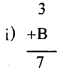Here : 3 + 4 = 7 ∴ B = 4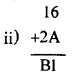Here 6 + A = 1 ∴ A = 5
6 + 5 = 11 is written in units place and 1 is carried.
∴ 1 + 1 + 2 = B
∴ B = 4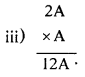Here A × A is A.
Hence A = 0, 1, 5, or 6 If A = 1 we get 21 × 1 = 21 but the product is 12A.
If A = 5 then 25 × 5 = 125.
∴ A = 5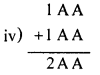Here A + A = A
Hence A = 0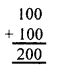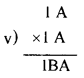Answer: Here A × A = A
Hence A = 0, 1, 5 or 6
If A = 1, then 11 × 11 = 121
∴ A = 1 and B = 2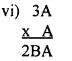3A × A
36 × 6
216
∴ A = 6 & B = 1

Question 2.
In the adjacent sum A, B, C are consecutive digits. In the third row A, B, C appear in some order. Find A, B, C.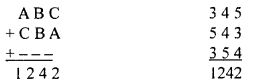∴ A = 3, B = 4 and C = 5

All Chapter KSEEB Solutions For Class 8 maths

—————————————————————————–

All Subject KSEEB Solutions For Class 9

*************************************************

I think you got complete solutions for this chapter. If You have any queries regarding this chapter, please comment on the below section our subject teacher will answer you. We tried our best to give complete solutions so you got good marks in your exam.

If these solutions have helped you, you can also share kseebsolutionsfor.com to your friends.

Best of Luck!!